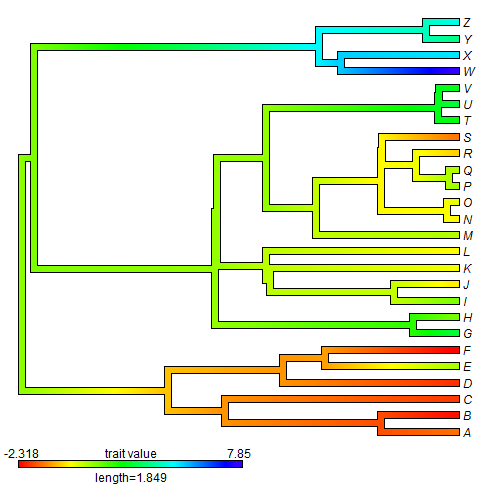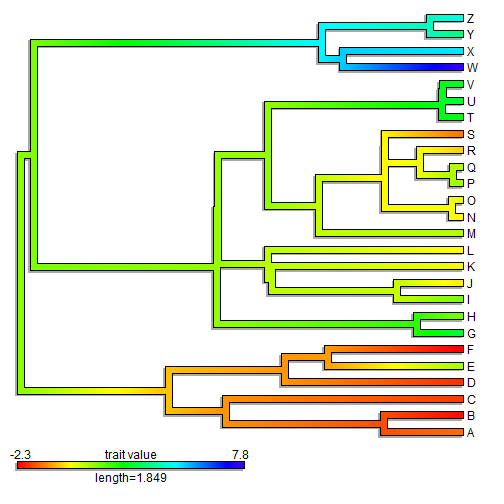## Thursday, May 25, 2017

### Add a 'shadow' visual effect to a plotted tree in R

Today I saw a cool visual effect in a plotted phytools object, although I suspect it was achieved by posthoc editing the plot in Adobe Illustrator rather than in R.

The effect was to add a 'shadow' to a plotted tree. Here is a quick demo of how to do it in R. I have illustrated the method using a plotted `"contMap"` object, because it is a little trickier, but we could do the same thing with any old plotted tree:

``````## load phytools
library(phytools)
## here is our tree & data
tree
``````
``````##
## Phylogenetic tree with 26 tips and 25 internal nodes.
##
## Tip labels:
##  A, B, C, D, E, F, ...
##
## Rooted; includes branch lengths.
``````
``````x
``````
``````##           A           B           C           D           E           F
## -1.28663899 -2.18638418 -1.76955549 -1.85871833  0.92086981 -2.31821640
##           G           H           I           J           K           L
##  3.04198683  1.41705375  1.35059487 -0.03275257  0.19923947  0.09906219
##           M           N           O           P           Q           R
##  0.84486447  0.06387185  0.27210453  1.02166697  0.85217792 -0.31925792
##           S           T           U           V           W           X
## -1.17999809  2.67084163  3.05705763  2.77059737  7.84958715  5.18219507
##           Y           Z
##  3.78072083  4.69439548
``````
``````## get our "contMap" object
obj<-contMap(tree,x,plot=FALSE,res=1000)
## now this is really just a test plot that we are going to
## compute to get our x & y limits
plot(obj,lwd=6)
````````````lastPP<-get("last_plot.phylo",envir=.PlotPhyloEnv)
## now our real plot:
par(fg="transparent")
plotTree(obj\$tree,color="darkgrey",lwd=8,xlim=lastPP\$x.lim,ylim=lastPP\$y.lim)
xlim<-lastPP\$x.lim-0.004*diff(lastPP\$x.lim)
ylim<-lastPP\$y.lim-0.004*diff(lastPP\$y.lim)
## this is not for the shadow, but to get an outline on our
## plotted contMap tree:
par(fg="black")
lines(c(0,0.5*max(nodeHeights(obj\$tree)))-0.004*diff(lastPP\$x.lim),
c(-1,-1)-0.004*diff(lastPP\$y.lim),lwd=8,lend=2,col="darkgrey")
title="trait value",prompt=FALSE,x=0,y=-1,lims=obj\$lims,lwd=6)
``````Pretty neat - I think.

The tree & data for this example were simulated as follows:

``````tree<-pbtree(n=26,tip.label=LETTERS)
x<-fastBM(tree)
``````